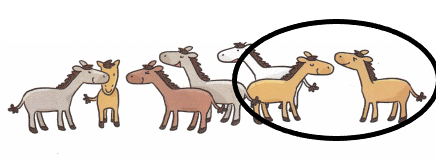# Math in Focus Kindergarten Chapter 4 Answer Key Counting and Numbers 0 to 10

Go through the Math in Focus Grade K Workbook Answer Key Chapter 4 Counting and Numbers 0 to 10 to finish your assignments.

## Math in Focus Kindergarten Chapter 4 Answer Key Counting and Numbers 0 to 10

Lesson 1 Composing and Decomposing 5

Count, write, and circle.

Question 1.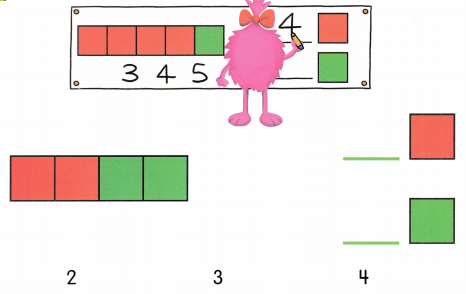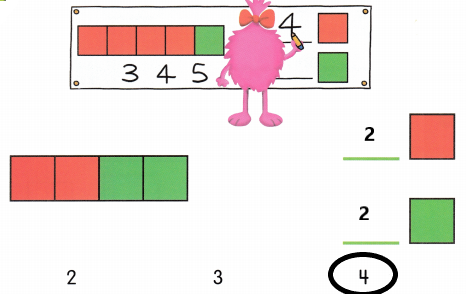Explanation:
There are 2 red squares and 2 green squares, 4 in all. So, i circled 4.

Question 2.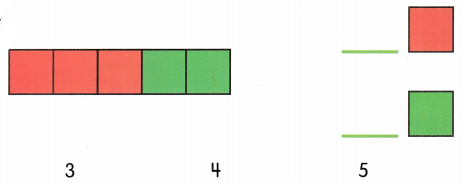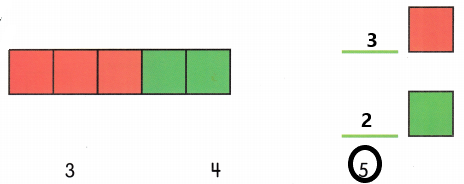Explanation:
There are 3 red squares and 2 green squares, 4 in all. So, i circled 5.

Color, count and write.

Question 1.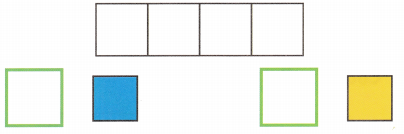4 is __________ and ___________.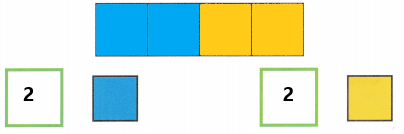Explanation:
I colored and wrote 2 blue squares and 2 yellow squares.

Question 2.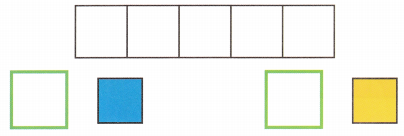5 is __________ and ___________.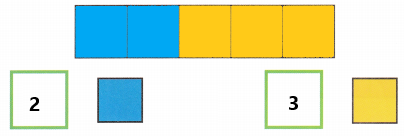Explanation:
I colored and wrote 2 blue squares and 3 yellow squares.

Question 3.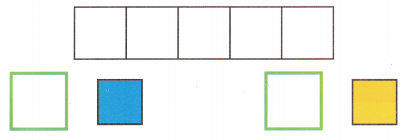5 is __________ and ___________.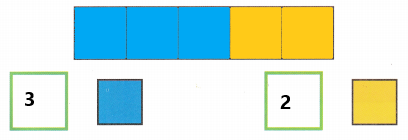Explanation:
I colored and wrote 2 blue squares and 2 yellow squares.

Lesson 2 Counting and Ordering Up to 10

Are there enough? Color.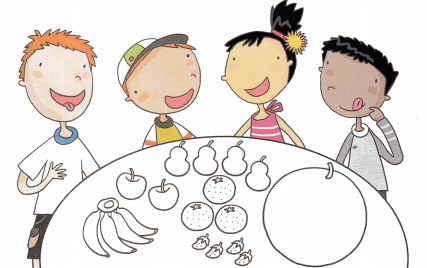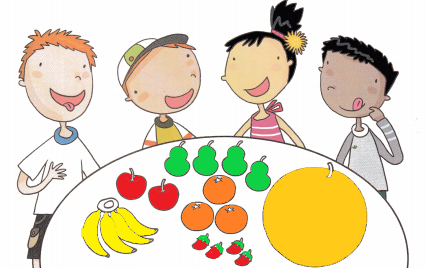Explanation:
There are 4 bananas, 4 strawberries and 4 pears.
The other fruits are not enough as they are less than four each.

Draw enough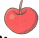. Count and write.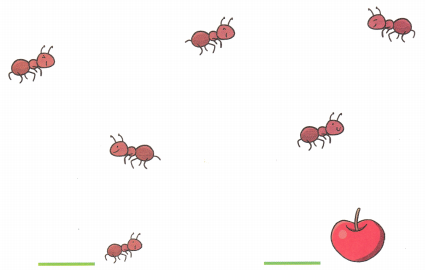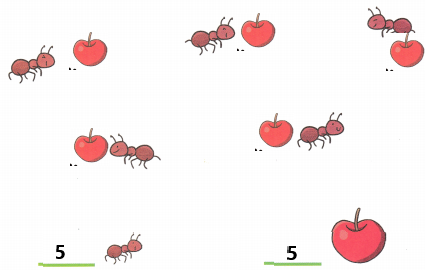Explanation:
I drew 5 apples and there are 5 ants and 5 apples.

Count and write.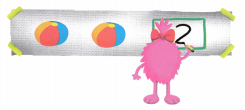Question 1.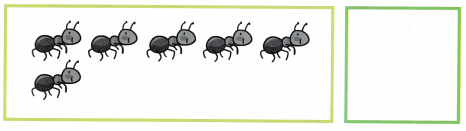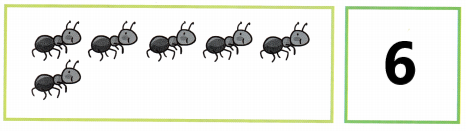Explanation:
I counted and wrote the number 6 as there are 6 ants.

Question 2.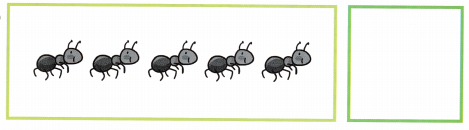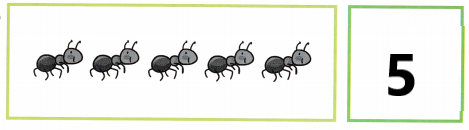Explanation:
I counted and wrote the number 5 as there are 5 ants.

Question 3.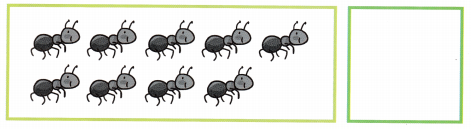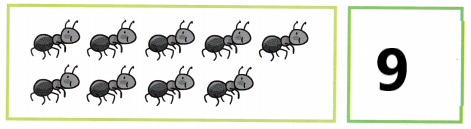Explanation:
I counted and wrote the number 9 as there are 9 ants.

Question 4.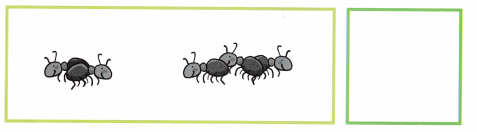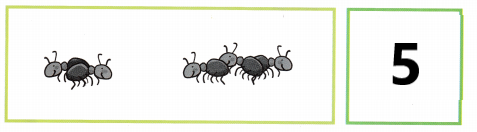Explanation:
I counted and wrote the number 5 as there are 5 ants.

Draw one more. Count and write.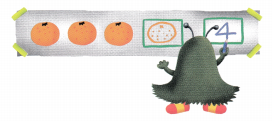Question 1.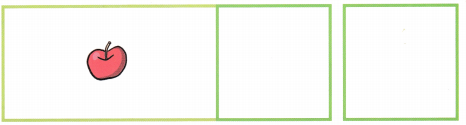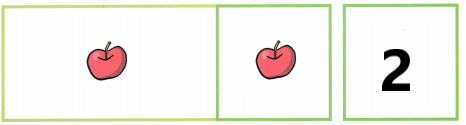Explanation:
I drew 1 more apple and i counted and wrote the number 2 as there are 2 apples.

Question 2.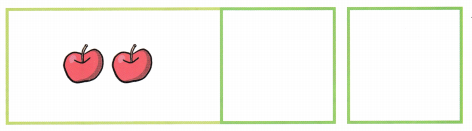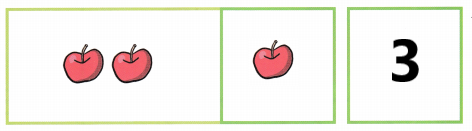Explanation:
I drew 1 more apple and i counted and wrote the number 3 as there are 3 apples.

Question 3.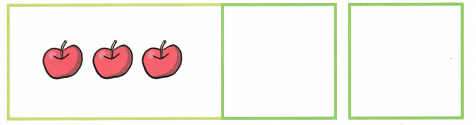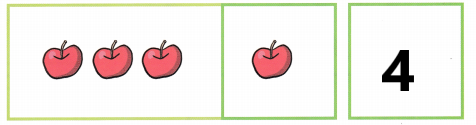Explanation:
I drew 1 more apple and i counted and wrote the number 4 as there are 4 apples.

Question 4.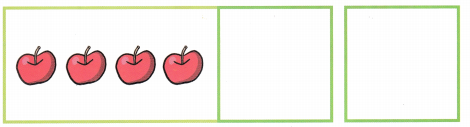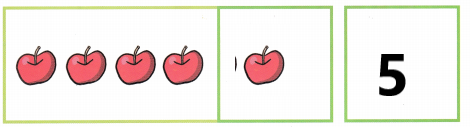Explanation:
I drew 1 more apple and i counted and wrote the number 5 as there are 5 apples.

Count and write.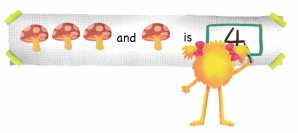Question 1.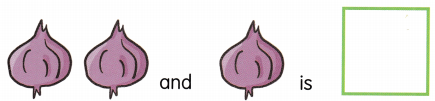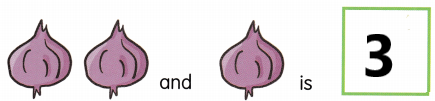Explanation:
I counted and wrote the number 3 as there are 3 turnips.

Question 2.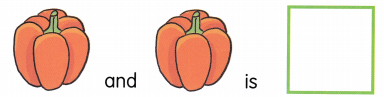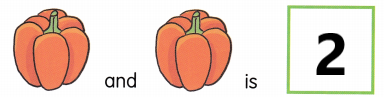Explanation:
I counted and wrote the number 2 as there are 2 capsicums.

Question 3.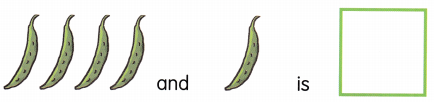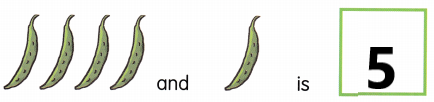Explanation:
I counted and wrote the number 5 as there are 5 green peas.

Question 4.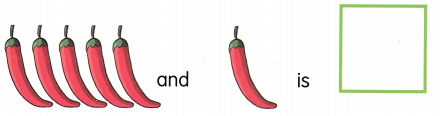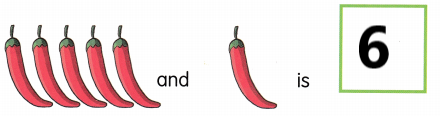Explanation:
I counted and wrote the number 6 as there are 6 chillies.

Draw, count and write.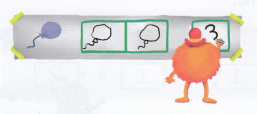Question 1.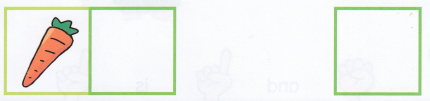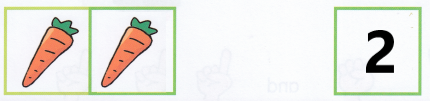Explanation:
I drew 1 more carrot and i counted and wrote the number 2.

Question 2.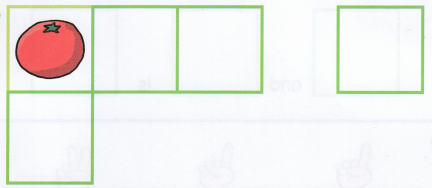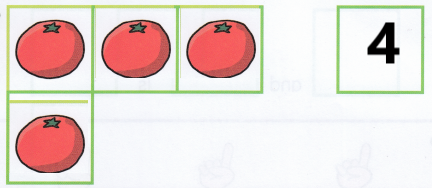Explanation:
I drew 3 more tomatoes and i counted and wrote the number 4.

Question 3.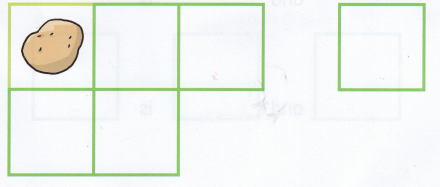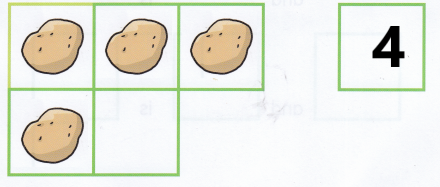Explanation:
I drew 3 more potatoes and i counted and wrote the number 4.

Lesson 3 Using Your Fingers and Toes to Count On

Count and write.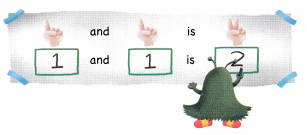Question 1.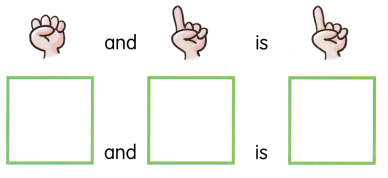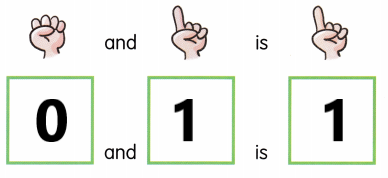Explanation:
I counted and wrote 0 and 1 is 1.

Question 2.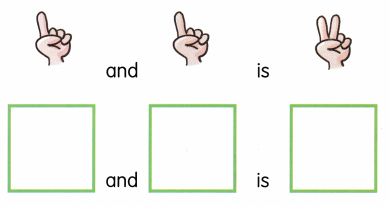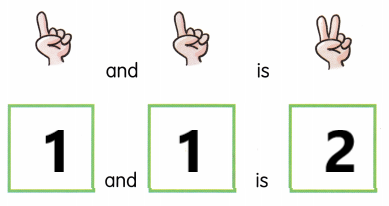Explanation:
I counted and wrote 1 and 1 is 2.

Question 3.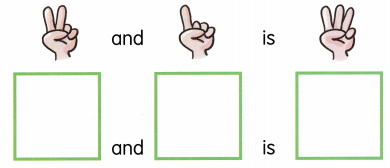Explanation:
I counted and wrote 2 and 1 is 3.

Question 4.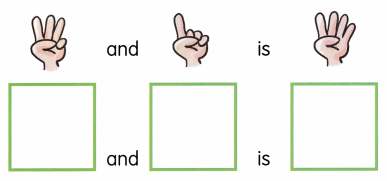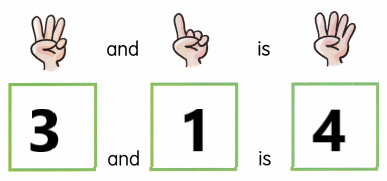Explanation:
I counted and wrote 3 an d1 is 4.

Question 5.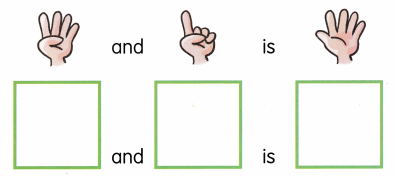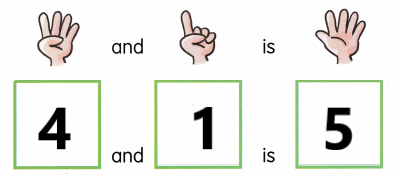Explanation:
I counted and wrote 4 and 1 is 5.

Lesson 4 Same Number and More

Look and talk.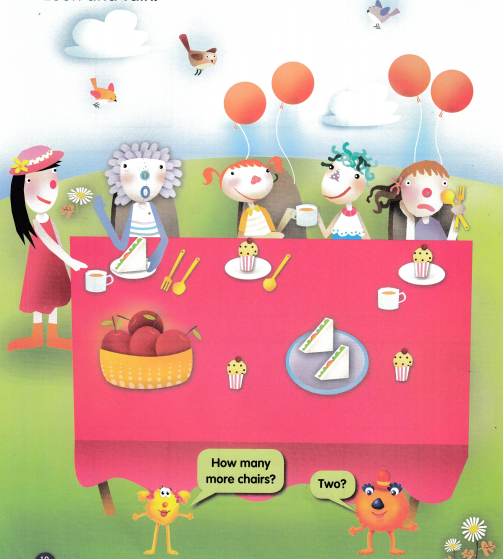How many more? Count and write.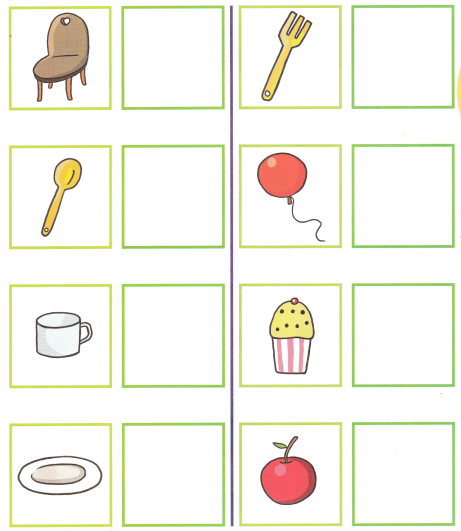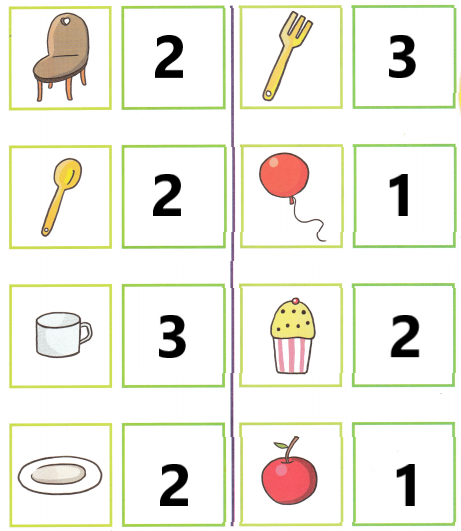Explanation:
I counted and wrote the numbers how many more are needed for 5 people.

Count and write.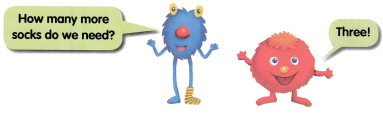Question 1.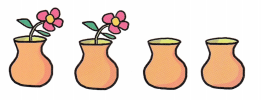__________ more flowers are needed.
2 more flowers are needed.

Question 2.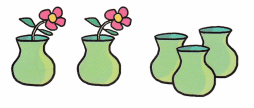__________ more flowers are needed.
3 more flowers are needed.

Question 3.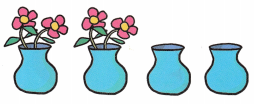__________ more flowers are needed.
4 more flowers are needed.

Lesson 5 Fewer Than

Circle.

Which group has fewer than 3?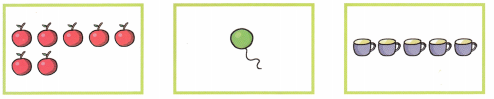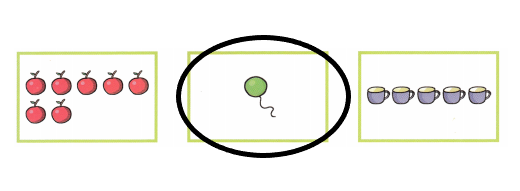Explanation:
I circled the group that has fewer than 3.

Which group has fewer than 5?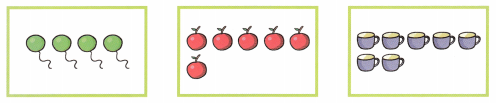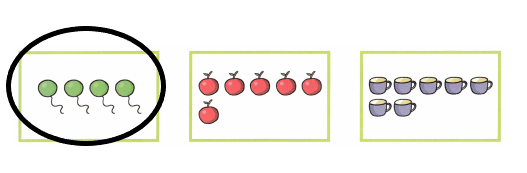Explanation:
I circled the group that has fewer than 5.

Which group has fewer than 7?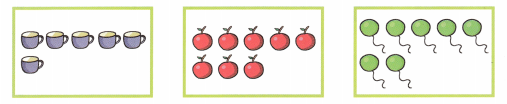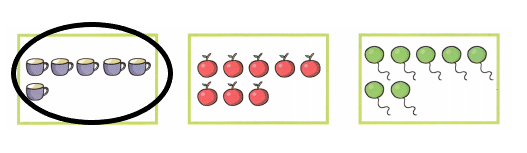Explanation:
I circled the group that has fewer than 7.

Which group has fewer than 9?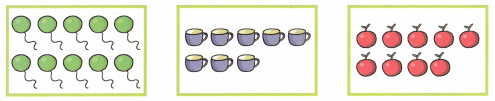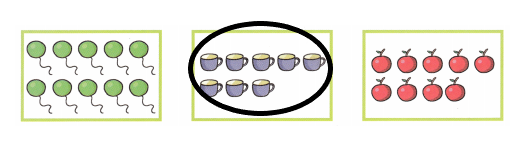Explanation:
I circled the group that has fewer than 9.

Lesson 6 How Many in All?

Draw one more. How many are there in all?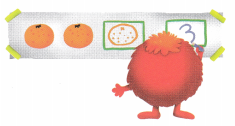Question 1.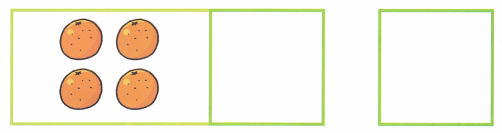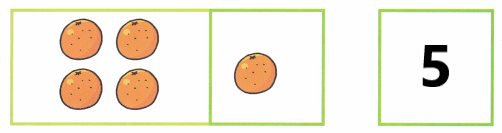Explanation:
I drew 1 more orange and i counted and wrote the number 5.

Question 2.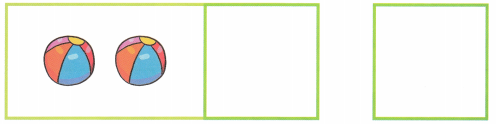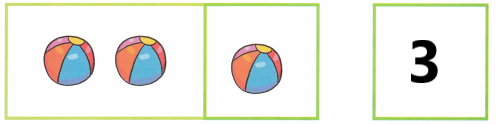Explanation:
I drew 1 more ball and i counted and wrote the number 3.

Question 3.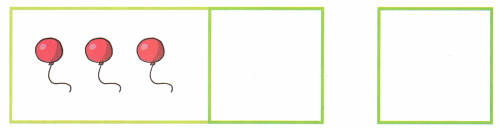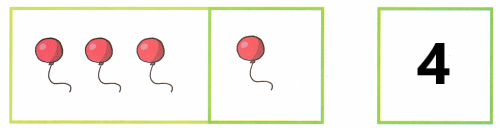Explanation:
I drew 1 more balloon and i counted and wrote the number 4.

Circle, count and write.

Question 1.
Vicki eats 2 grapes. Circle the grapes that are left behind.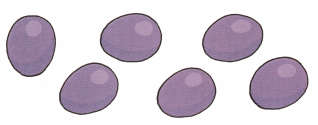___________ grapes are left behind.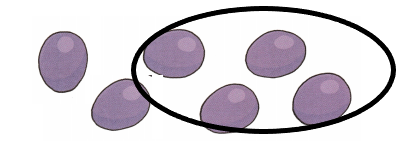Explanation:
4 grapes are left behind. So, i circled 4 grapes.

Question 2.
2 birds fly away. Circle the birds that stay behind.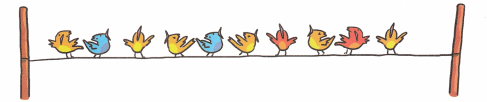___________ birds stay behind.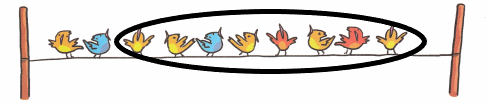Explanation:
8 birds stay behind. So, i circled 8 birds.

Question 3.
4 horses trot away. Circle the horses that stay behind.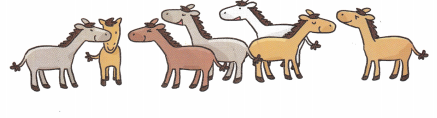____________ horses stay behind.